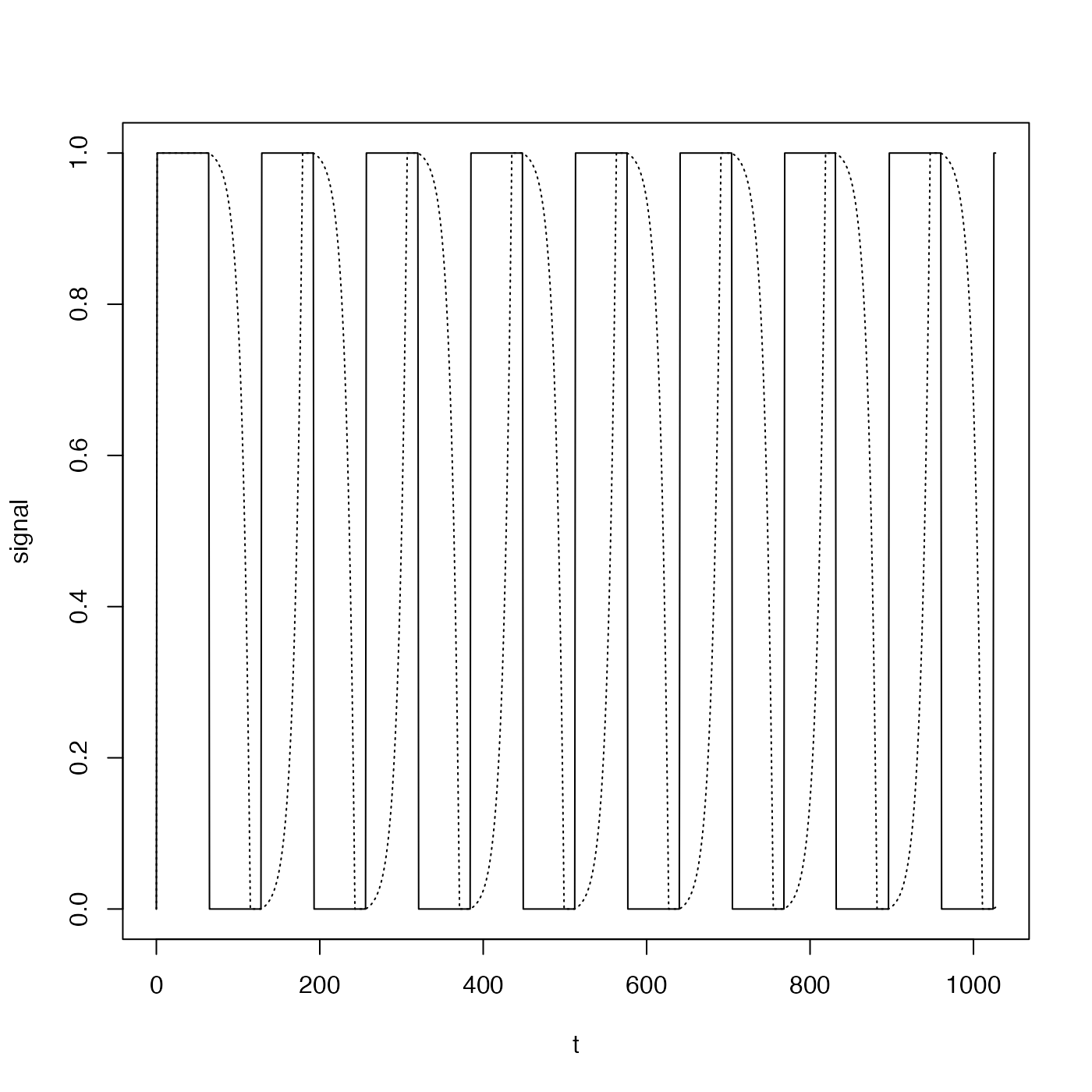Convolve two time series, using a backward-looking method. This function provides a straightforward convolution, which may be useful to those who prefer not to use convolve() and filter in the stats package.

oceConvolve(x, f, end = 2)

## Arguments

x a numerical vector of observations. a numerical vector of filter coefficients. a flag that controls how to handle the points of the x series that have indices less than the length of f. If end=0, the values are set to 0. If end=1, the original x values are used there. If end=2, that fraction of the f values that overlap with x are used.

## Value

A vector of the convolution output.

## Examples

library(oce)
t <- 0:1027
n <- length(t)
signal <- ifelse(sin(t * 2 * pi / 128) > 0, 1, 0)
tau <- 10
filter <- exp(-seq(5*tau, 0) / tau)
filter <- filter / sum(filter)
observation <- oce.convolve(signal, filter)
plot(t, signal, type='l')lines(t, observation, lty='dotted')